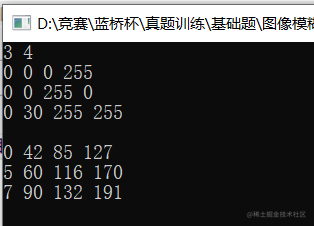## 算法题每日一练---第4天：图像模糊问题

「这是我参与2022首次更文挑战的5天，活动详情查看：2022首次更文挑战

## 四、编码实现

``````#include<iostream>
using namespace std;
int main()
{
int a,b;//定义两个二维数组
int c={0,1,0,-1,-1,0,1,0,1,1,-1,1,-1,-1,1,-1};//方向数组
int n,m,i,j,k;
cin>>n>>m;//输入数据
for(i=1;i<=n;i++)
{
for(j=1;j<=m;j++)
{
cin>>a[i][j];//输入
}
}
int ans,sum=0;//定义，周围的像素和和个数
for(i=1;i<=n;i++)
{
for(j=1;j<=m;j++)
{
sum=a[i][j];//像素值初始化
ans=1;//像素个数初始化
for(k=0;k<8;k++)
{
int x=i+c[k];//x坐标
int y=j+c[k];//y坐标
if(x>=1&&x<=n&&y>=1&&y<=m)//判断坐标是否在范围
{
ans++;//像素个数++
sum+=a[x][y];//像素值++
}
}
b[i][j]=sum/ans;//更新变量值
}
}
for(i=1;i<=n;i++)
{
for(j=1;j<=m;j++)
{
cout<<b[i][j]<<" ";//输出数组
}cout<<"\n";
}
return 0;
}

## 四、输出结果

3 4

0 0 0 255

0 0 255 0

0 30 255 255